• 数据标准化进行随机森林分类
千次阅读
2021-11-06 16:22:47

## 机器学习一：使用决策树和随机森林对数据分类

首先导入数据：income_classification.csv
在python编辑器中导入所需要的库

import numpy as np
import pandas as pd
from sklearn import tree
from sklearn.ensemble import RandomForestClassifier
from sklearn.feature_extraction import DictVectorizer
from sklearn.preprocessing import LabelEncoder



## 题目一：读入数据并显示数据的维度和前5行数据。

#题目一：读入数据并显示数据的维度和前五行数据
print('1.载入数据.....')
print('数据的维度和前五行数据：', data.shape)



## 题目二：对连续变量age进行离散化处理并显示前五行。

print('对连续变量age进行离散化处理，等宽分成五类，显示前五行：')
AGE_CUT = pd.cut(x=data['age'], bins=5, labels=range(0, 5))
data['age'] = AGE_CUT


## 题目三：对属性是字符串的任意特征进行数字编号处理，显示前5行编码后的结果，每个特定的字符串用一个整数来表示，整数序列从0开始增长。

class_le = LabelEncoder()
data['workclass'] = pd.DataFrame(class_le.fit_transform(data['workclass']))
data['marital-status'] = pd.DataFrame(class_le.fit_transform(data['marital-status']))
data['occupation'] = pd.DataFrame(class_le.fit_transform(data['occupation']))
data['education'] = pd.DataFrame(class_le.fit_transform(data['education']))
data['native-country'] = pd.DataFrame(class_le.fit_transform(data['native-country']))
data['relationship'] = pd.DataFrame(class_le.fit_transform(data['relationship']))
data['race'] = pd.DataFrame(class_le.fit_transform(data['race']))
data['sex'] = pd.DataFrame(class_le.fit_transform(data['sex']))
print('显示前五行编码后的结果：')


## 题目四：对预处理后的数据用决策树算法和随机森林算法分类。

实验步骤

1. 选择合适的若干特征字段
2. 按7:3划分训练集和样本集
3. 使用训练集训练一个决策树分类器
4. 使用测试集计算决策树分类器的分类准确率
5. 使用训练集训练一个随机森林分类器
6. 使用测试集计算随机森林分类器的分类准确率
data1 = []
labels = []
for index, row in data.iterrows():
# data需要是字典形式，因为之后需要使用DictVectorizer()修改字符串数据类型，以便符合DecisionTreeClassifier()
rowDict = {}
row = list(row)
rowDict['age'] = row
rowDict['workclass'] = row
rowDict['education'] = row
rowDict['education_num'] = row
rowDict['maritial_status'] = row
rowDict['occupation'] = row
rowDict['relationship'] = row
rowDict['race'] = row
rowDict['sex'] = row
rowDict['capital_gain'] = row
rowDict['capital_loss'] = row
rowDict['hours_per_week'] = row
rowDict['native_country'] = row
data1.append(rowDict)
labels.append(row[-1])

print('2. 构造数据和标签.....')
x = np.array(data1)
labels = np.array(labels)
y = np.zeros(labels.shape)  # 初始label全为0
y[labels == '<=50K'] = 0  # 当label等于这三种属性的话，设置为1。
y[labels == '>50K'] = 1

# 转换字符串数据类型
print('3.转换字符串数据类型.....')
vec = DictVectorizer()
dx = vec.fit_transform(x).toarray()

# 拆分成训练数据和测试数据
print('4.拆分训练数据和测试数据.....')
print('训练集和验证集比例7:3')
ratio = 0.7
xTrain = []
yTrain = []
xTest = []
yTest = []
features = xTrain, xTest
labels = yTrain, yTest
for i in range(len(dx)):
dataSetIndex = 0 if np.random.random() < ratio else 1
features[dataSetIndex].append(dx[i])
labels[dataSetIndex].append(y[i])

# CART决策树分类
print('5.CART决策树分类.....')
clf_cart = tree.DecisionTreeClassifier(criterion='entropy')  # CART算法，使用entropy作为标准；默认是是用gini作为标准
clf_cart.fit(xTrain, yTrain)

# 检查准确率
accuracy_cart = clf_cart.score(xTest, yTest)
print('CART树分类准确率：', accuracy_cart)

print('6.随机森林分类.....')
clf_random = RandomForestClassifier()
clf_random.fit(xTrain, yTrain)

# 检查准确率
accuracy_random = clf_random.score(xTest, yTest)
print('随机森林分类准确率：', accuracy_random)决策树 机器学习
更多相关内容
• “实践是检验真理的唯一标准。”“复现是学习生信的最好办法。”2021.4.12_1DOI: 10.1016/j.cell.2020.05.032这篇2020年发表在cell上关于新冠的组学...

“实践是检验真理的唯一标准。”

“复现是学习生信的最好办法。”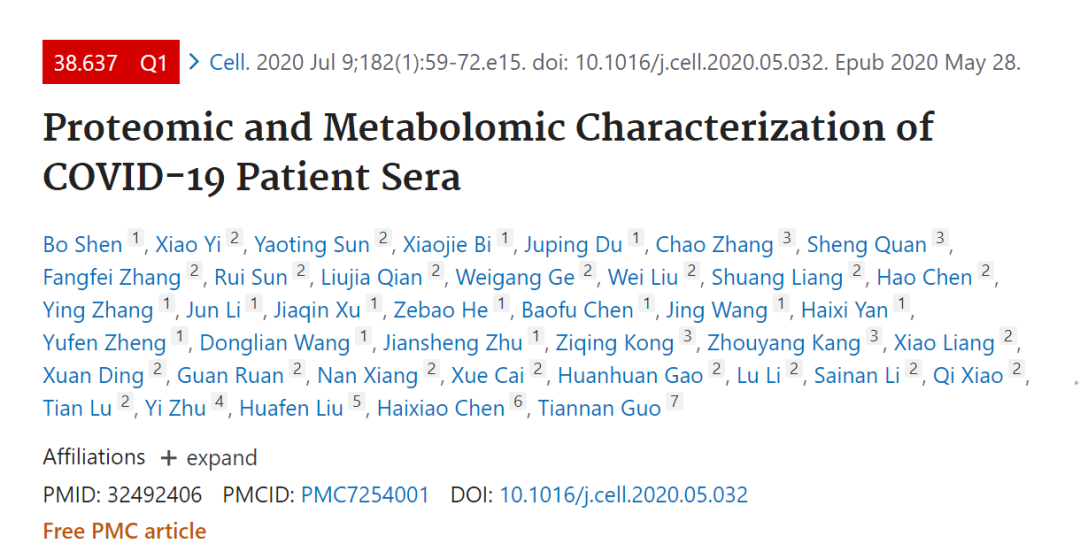DOI: 10.1016/j.cell.2020.05.032

这篇2020年发表在cell上关于新冠的组学文章里面有大量的生信内容。今天带大家复现其中的一个分析：随机森林分类模型的构建以及对重要变量的选择及其简约模型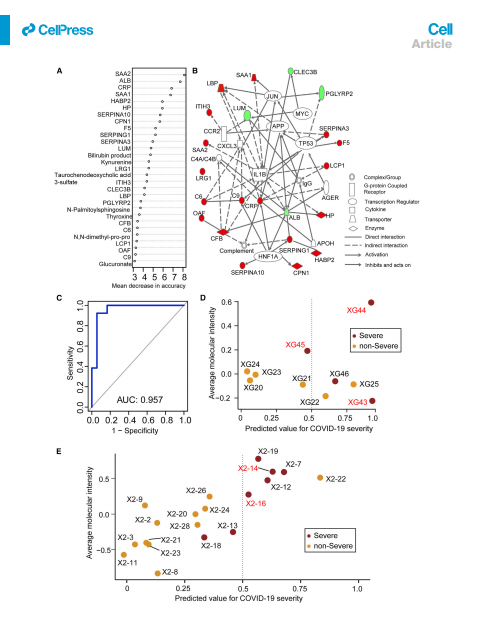• 随机森林简介

• 数据准备

• 数据预处理

• 构建随机森林模型

• 训练集测试

• 训练集分类可视化(复现图2D)

• 绘制训练集ROC

• 使用测试集评估

• 训练集分类可视化

• 测试集ROC

• 十折交叉验证

• 特征选取(复现2A)

• 简约随机森林分类模型的构建

• 训练集测试及分类可视化

• 测试集测试及分类可视化

• 代码数据获取及微信答疑群

• 往期内容

本文内容干货较多(12000字），所以尝试一下付费。文末有微信交流群，交流科研相关问题。

## 随机森林简介

展开全文机器学习 人工智能
• oob_score三、重要属性和接口四、随机森林回归器1、重要参数，属性与接口2、实例：用随机森林回归填补缺失值五、机器学习中调参的基本思想六、 实例：随机森林在乳腺癌数据上的调参 一、集成算法概述 集成学习...

# 一、集成算法概述

集成学习（ensemble learning）是时下非常流行的机器学习算法，它本身不是一个单独的机器学习算法，而是通过在数据上构建多个模型，集成所有模型的建模结果。基本上所有的机器学习领域都可以看到集成学习的身影，在现实中集成学习也有相当大的作用，它可以用来做市场营销模拟的建模，统计客户来源，保留和流失，也可用来预测疾病的风险和病患者的易感性。在现在的各种算法竞赛中，随机森林，梯度提升树（GBDT），Xgboost等集成 算法的身影也随处可见，可见其效果之好，应用之广。

多个模型集成成为的模型叫做集成评估器（ensemble estimator），组成集成评估器的每个模型都叫做基评估器 （base estimator）。通常来说，有三类集成算法：装袋法（Bagging），提升法（Boosting）和stacking。

**装袋法的核心思想是构建多个相互独立的评估器，然后对其预测进行平均或多数表决原则来决定集成评估器的结 果。装袋法的代表模型就是随机森林。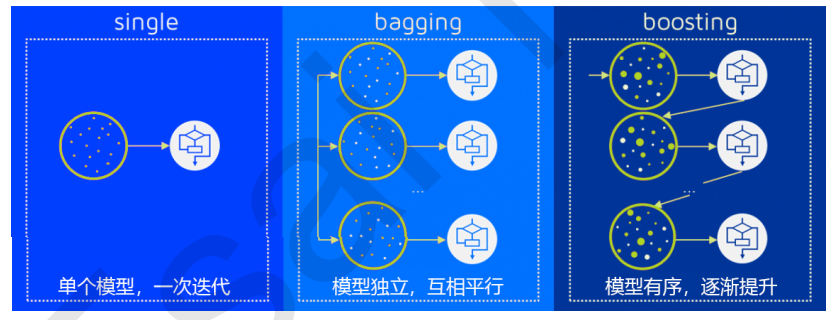# 二、重要参数

## 1、控制基评估器的参数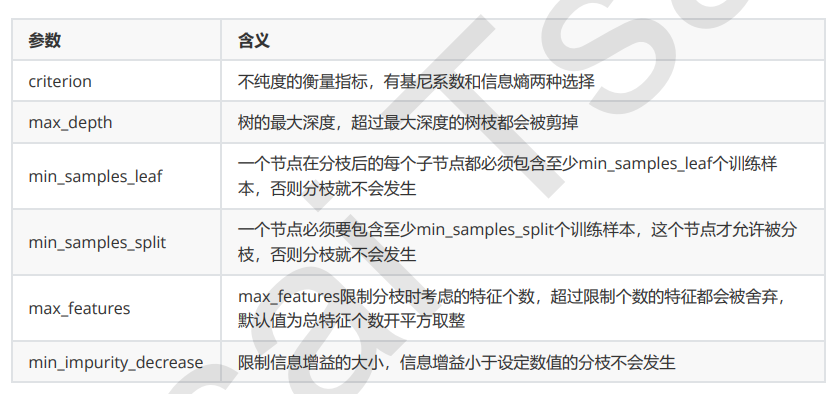单个决策树的准确率越高，随机森林的准确率也会越高，因为装袋法是依赖于平均值或 者少数服从多数原则来决定集成的结果的。

## 2、n_estimators

n_estimators越 大，模型的效果往往越好。但是相应的，任何模型都有决策边界，n_estimators达到一定的程度之后，随机森林的 精确性往往不在上升或开始波动，并且，n_estimators越大，需要的计算量和内存也越大，训练的时间也会越来越 长。对于这个参数，我们是渴望在训练难度和模型效果之间取得平衡。

n_estimators的默认值在现有版本的sklearn中是10，但是在即将更新的0.22版本中，这个默认值会被修正为 100。这个修正显示出了使用者的调参倾向：要更大的n_estimators。

from sklearn.tree import DecisionTreeClassifier
from sklearn.ensemble import RandomForestClassifier
from sklearn.model_selection import train_test_split
from sklearn.model_selection import cross_val_score
from matplotlib import pyplot as plt

x_train, x_test, y_train, y_test = train_test_split(wine.data,wine.target, test_size=0.3)

rfc = RandomForestClassifier(n_estimators=25)
rfc = rfc.fit(x_train, y_train)
score = rfc.score(x_test, y_test)
print(score)
#0.9814814814814815


画出随机森林和决策树在一组交叉验证下的效果对比

rfc = RandomForestClassifier(n_estimators=25)
rfc_s = cross_val_score(rfc,wine.data,wine.target,cv=10)
clf = DecisionTreeClassifier()
clf_s = cross_val_score(clf,wine.data,wine.target,cv=10)
plt.plot(range(1,11),rfc_s,label = "RandomForest")
plt.plot(range(1,11),clf_s,label = "Decision Tree")
plt.legend()
plt.show()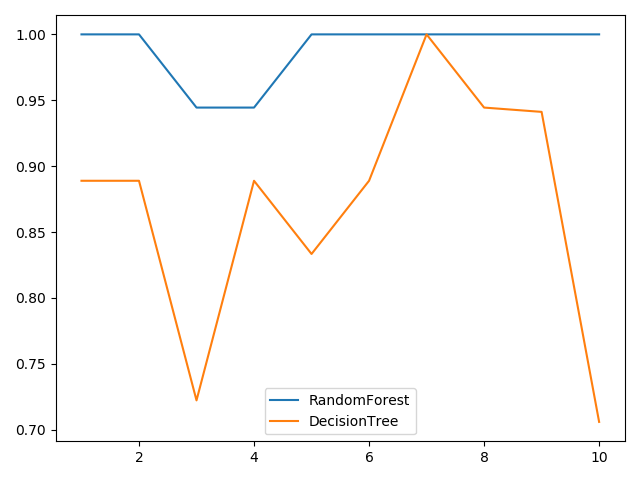画出随机森林和决策树在十组交叉验证下的效果对比

rfc_l = []
clf_l = []
for i in range(10):
rfc = RandomForestClassifier(n_estimators=25)
rfc_s = cross_val_score(rfc,wine.data,wine.target,cv=10).mean()
rfc_l.append(rfc_s)
clf = DecisionTreeClassifier()
clf_s = cross_val_score(clf,wine.data,wine.target,cv=10).mean()
clf_l.append(clf_s)

plt.plot(range(1,11),rfc_l,label = "Random Forest")
plt.plot(range(1,11),clf_l,label = "Decision Tree")
plt.legend()
plt.show()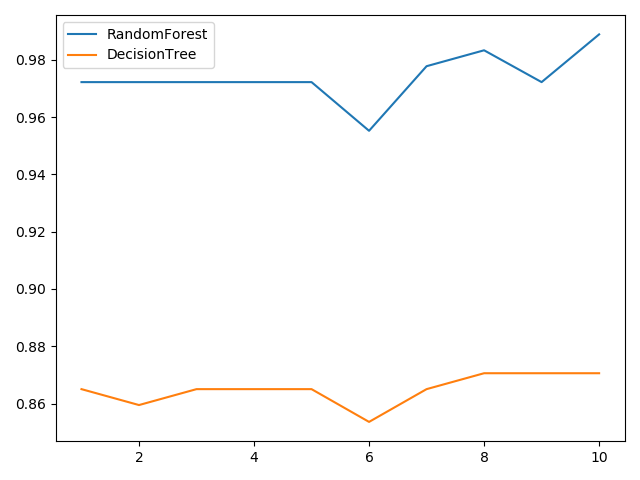n_estimators的学习曲线

superpa = []
for i in range(200):
rfc = RandomForestClassifier(n_estimators=i+1, n_jobs=-1)
rfc_s = cross_val_score(rfc, wine.data, wine.target, cv=10).mean()
superpa.append(rfc_s)
print(max(superpa), superpa.index(max(superpa)))
#0.9888888888888889 23
plt.figure(figsize=[20, 5])
plt.plot(range(1, 201), superpa)
plt.show()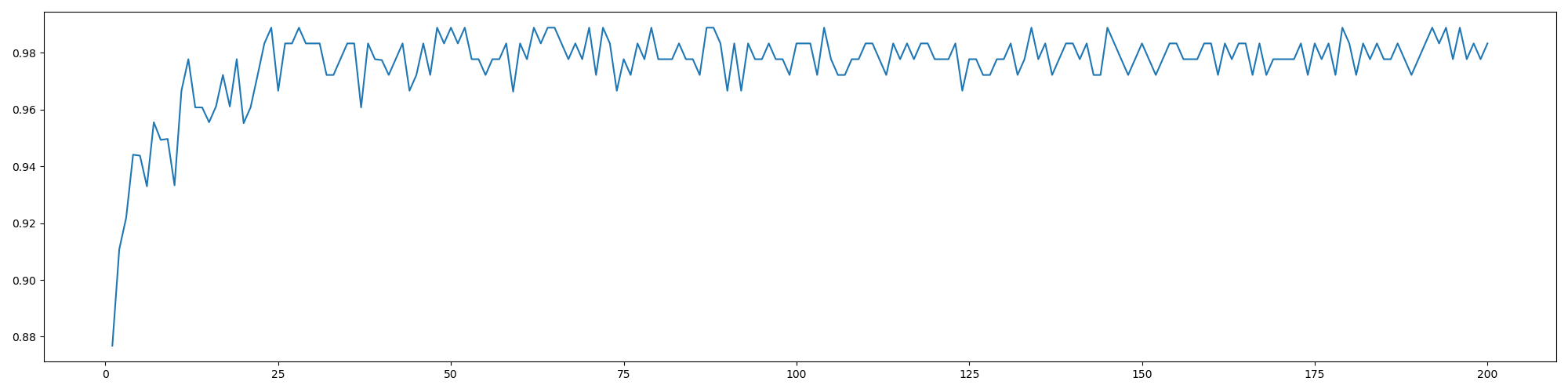## 3、random_state

随机森林的本质是一种装袋集成算法（bagging），装袋集成算法是对基评估器的预测结果进行平均或用多数表决原则来决定集成评估器的结果。在刚才的红酒例子中，我们建立了25棵树，对任何一个样本而言，平均或多数表决原则下，当且仅当有13棵以上的树判断错误的时候，随机森林才会判断错误。单独一棵决策树对红酒数据集的分类准确率在0.85上下浮动，假设一棵树判断错误的可能性为0.2(ε)，那20棵树以上都判断错误的可能性是：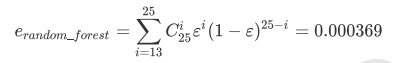可见，判断错误的几率非常小，这让随机森林在红酒数据集上的表现远远好于单棵决策树。

随机森林中其实也有random_state，用法和分类树中相似，只不过在分类树中，一个random_state只控制生成一 棵树，而随机森林中的random_state控制的是生成森林的模式，而非让一个森林中只有一棵树。

当random_state固定时，随机森林中生成是一组固定的树，但每棵树依然是不一致的，这是 用”随机挑选特征进行分枝“的方法得到的随机性。并且我们可以证明，当这种随机性越大的时候，袋装法的效果一 般会越来越好。用袋装法集成时，基分类器应当是相互独立的，是不相同的。

## 4、bootstrap & oob_score

要让基分类器尽量都不一样，一种很容易理解的方法是使用不同的训练集来进行训练，而袋装法正是通过有放回的随机抽样技术来形成不同的训练数据，bootstrap就是用来控制抽样技术的参数。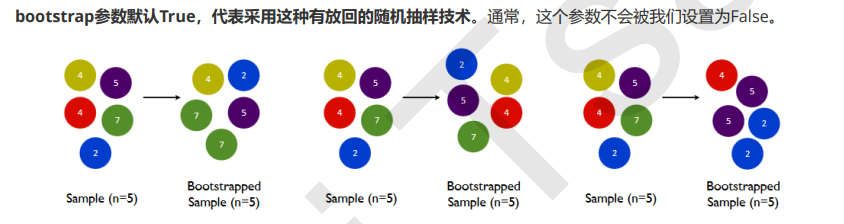一般来说，自助集大约平均会包含63%的原始数据。因为每一个样本被抽到某个自助集中的概率为：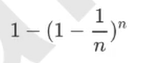当n足够大时，这个概率收敛于1-(1/e)，约等于0.632。因此，会有约37%的训练数据被浪费掉，没有参与建模， 这些数据被称为袋外数据(out of bag data，简写为oob)。

也就是说，在使用随机森林时，我们可以不划分测试集和训练集，只需要用袋外 数据来测试我们的模型即可。

#无需划分训练集和测试集然后进行交叉验证，也可以不划分，直接观察袋外数据的测试分数
rfc = RandomForestClassifier(n_estimators=25, oob_score=True)
rfc = rfc.fit(wine.data, wine.target)
#重要属性oob_score_
print(rfc.oob_score_)
#0.9662921348314607


# 三、重要属性和接口

除了.estimators_ 和 .oob_score_ 这两个重要属性。随机森林自然也有.feature_importances_这个属性。随机森林的接口与决策树完全一致，因此依然有四个常用接口：apply, fit, predict和score。除此之外，还需要注意随机森林的predict_proba接口，这个接口返回每个测试样本对应的被分到每一类标签的概率，标签有几个分类就返回几个概率。则predict_proba返回的数值大于0.5的，被分为1，小于0.5的，被分为0

rfc = RandomForestClassifier(n_estimators=25)
rfc = rfc.fit(x_train, y_train)
score = rfc.score(x_test, y_test)
print(score)
#0.9814814814814815

print(rfc.feature_importances_)  #得到所有特征值的重要性系数
print(rfc.apply(x_test))  #得到测试集所被分配到的叶子结点
rfc.predict(Xtest)
rfc.predict_proba(Xtest)


Bonus：Bagging的另一个必要条件
之前我们说过，在使用袋装法时要求基评估器要尽量独立。其实，袋装法还有另一个必要条件：基分类器的判断准确率至少要超过随机分类器，即时说，基分类器的判断准确率至少要超过50%。

import numpy as np
x = np.linspace(0,1,20)
y = []
for epsilon in np.linspace(0,1,20):
E = np.array([comb(25,i)*(epsilon**i)*((1-epsilon)**(25-i))
for i in range(13,26)]).sum()
y.append(E)
plt.plot(x,y,"o-",label="when estimators are different")
plt.plot(x,x,"--",color="red",label="if all estimators are same")
plt.xlabel("individual estimator's error")
plt.ylabel("RandomForest's error")
plt.legend()
plt.show()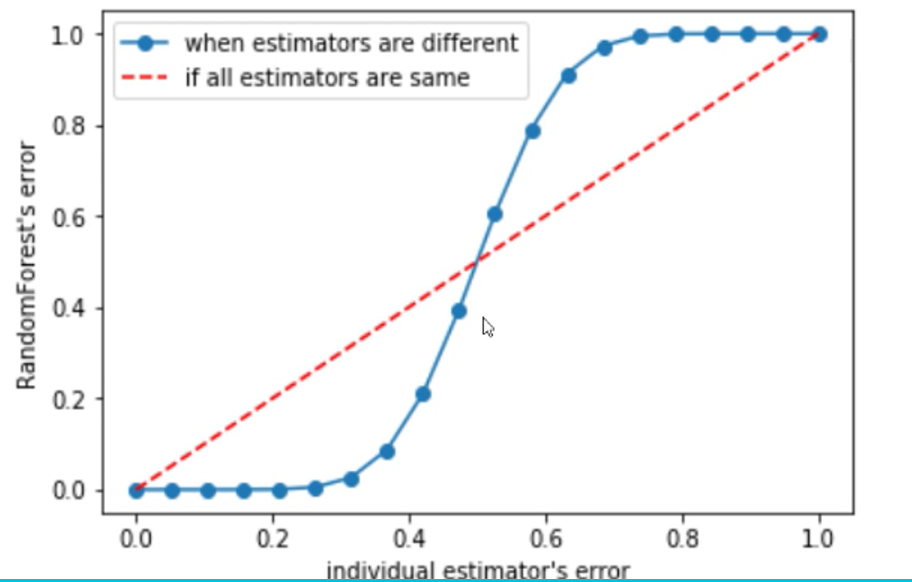# 四、随机森林回归器

## 1、重要参数，属性与接口

criterion

回归树衡量分枝质量的指标，支持的标准有三种：
1）输入"mse"使用均方误差mean squared error(MSE)，父节点和叶子节点之间的均方误差的差额将被用来作为 特征选择的标准，这种方法通过使用叶子节点的均值来最小化L2损失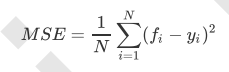2）输入“friedman_mse”使用费尔德曼均方误差，这种指标使用弗里德曼针对潜在分枝中的问题改进后的均方误差

3）输入"mae"使用绝对平均误差MAE（mean absolute error），这种指标使用叶节点的中值来最小化L1损失

在回归中，我们追求的是，MSE越小越好。 然而，回归树的接口score返回的是R平方，并不是MSE。R平方被定义如下：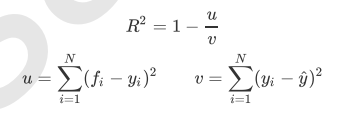虽然均方误差永远为正，但是sklearn当中使用均方误差作为评判标准时，却是计算”负均方误 差“（neg_mean_squared_error）。

最重要的属性和接口，都与随机森林的分类器相一致，还是apply, fit, predict和score最为核心。值得一提的是，随 机森林回归并没有predict_proba这个接口，因为对于回归来说，并不存在一个样本要被分到某个类别的概率问 题，因此没有predict_proba这个接口。

## 2、实例：用随机森林回归填补缺失值

我们从现实中收集的数据，几乎不可能是完美无缺的，往往都会有一些缺失值。面对缺失值，很多人选择的方式是 直接将含有缺失值的样本删除，这是一种有效的方法，但是有时候填补缺失值会比直接丢弃样本效果更好，即便我 们其实并不知道缺失值的真实样貌。我们可以使用sklearn.impute.SimpleImputer来轻松地将均 值，中值，或者其他最常用的数值填补到数据中

import numpy as np
import pandas as pd
import matplotlib.pyplot as plt
from sklearn.ensemble import RandomForestRegressor
from sklearn.impute import SimpleImputer
from sklearn.model_selection import cross_val_score

#print(boston)
x = boston.data
y = boston.target

#print(x.shape)
#(506, 13)
#print(y.shape)
#(506,)

x_full, y_full = x, y
n_samples = x_full.shape
n_features = x_full.shape

#制造缺失值
rng = np.random.RandomState(0)
missing_rate = 0.5
n_missing_samples = int(np.floor(n_samples * n_features * missing_rate))
#np.floor向下取整，返回.0格式浮点数

#所有数据要随机遍布在数据集的各行各列当中，而一个缺失的数据会需要一个行索引和一个列索引
#如果能够创造一个数组，包含3289个分布在0~506中间的行索引，和3289个分布在0~13之间的列索引，那我们就可以利用索引来为数据中的任意3289个位置赋空值
#然后我们用0，均值和随机森林来填写这些缺失值，然后查看回归的结果如何

missing_features = rng.randint(0, n_features, n_missing_samples)
missing_samples = rng.randint(0, n_samples, n_missing_samples)
#randint(起始值，终止值，产生的随机数个数）

x_missing = x_full.copy()
y_missint = y_full.copy()

x_missing[missing_samples, missing_features] = np.nan
#print(x_missing)

x_missing = pd.DataFrame(x_missing)
#print(x_missing)

#使用0和均值填补缺失值
imp_mean = SimpleImputer(missing_values=np.nan, strategy='mean')
x_missing_mean = imp_mean.fit_transform(x_missing)
#print(x_missing_mean)

#用零来填补
imp_0 = SimpleImputer(missing_values=np.nan, strategy='constant', fill_value=0)
#strategy='constant'r————>填补一个常数，数值为0
x_missing_0 = imp_mean.fit_transform(x_missing)
#print(x_missing_0)

#用随机森林回归来填补缺失值
x_missing_reg = x_missing.copy()
#print(x_missing.isnull().sum(axis=0)) 可以得到每隔列索引上的缺失值个数，但我们要的是缺失值从小到大排列的索引顺序
sort_values = np.sort(x_missing.isnull().sum(axis=0))
#print(sort_index)  #[185 189 196 197 197 200 200 201 201 202 203 204 214]得到的是缺失值个数的排列，这还不是想要的，我们要的是索引值
sort_index = np.argsort(x_missing.isnull().sum(axis=0))
#print(sort_index)  #得到了所有列缺失值个数从小到大的排列顺序，接下来要从缺失值最小的列开始，也就是按照sort_index里面的顺序开始

#建立一个新的pf_0，为了不改变原有的x_missing_reg数值

#print(pf_0) #是datafram格式

for i in sort_index:
# 构建我们的新特征矩阵和新标签
df = x_missing_reg.copy()
fillc = df.iloc[:, i]
df = pd.concat([df.iloc[:, df.columns != i], pd.DataFrame(y_full)], axis=1)

# 在新特征矩阵中，对含有缺失值的列，进行0的填补
df_0 = SimpleImputer(missing_values=np.nan,
strategy='constant', fill_value=0).fit_transform(df)

# 找出我们的训练集和测试集
Ytrain = fillc[fillc.notnull()]
Ytest = fillc[fillc.isnull()]
Xtrain = df_0[Ytrain.index, :]
Xtest = df_0[Ytest.index, :]

# 用随机森林回归来填补缺失值
rfc = RandomForestRegressor(n_estimators=100)
rfc = rfc.fit(Xtrain, Ytrain)
Ypredict = rfc.predict(Xtest)

#将填补好的特征返回到原始特征矩阵中
x_missing_reg.loc[x_missing_reg.iloc[:, i].isnull(), i] = Ypredict

#对填补好的数据进行建模
#对所有数据进行建模，取得MSE结果
X = [x_full, x_missing_mean, x_missing_0, x_missing_reg]

mse = []
for x in X:
estimator = RandomForestRegressor(n_estimators=100, random_state=0)
scorces = cross_val_score(estimator, x, y_full, cv=5, scoring='neg_mean_squared_error').mean()

mse.append(scorces * -1)

#用所得的结果画图
x_labels = ['full data', 'zero imputation', 'mean imputation', 'regressor imputation']
color = ['r', 'g', 'b', 'orange']
plt.figure(figsize=(12, 6))
ax = plt.subplot(111)
for i in range(len(x_labels)):
ax.barh(i, mse[i], color=color[i], alpha=0.6, align='center')

ax.set_title('Imputation Techniques with Boston Data')
ax.set_xlim(left=np.min(mse) * 0.9, right=np.max(mse) * 1.1)
ax.set_yticks(np.arange(len(mse)))
ax.set_xlabel('MSE')
ax.set_yticklabels(x_labels)
plt.show()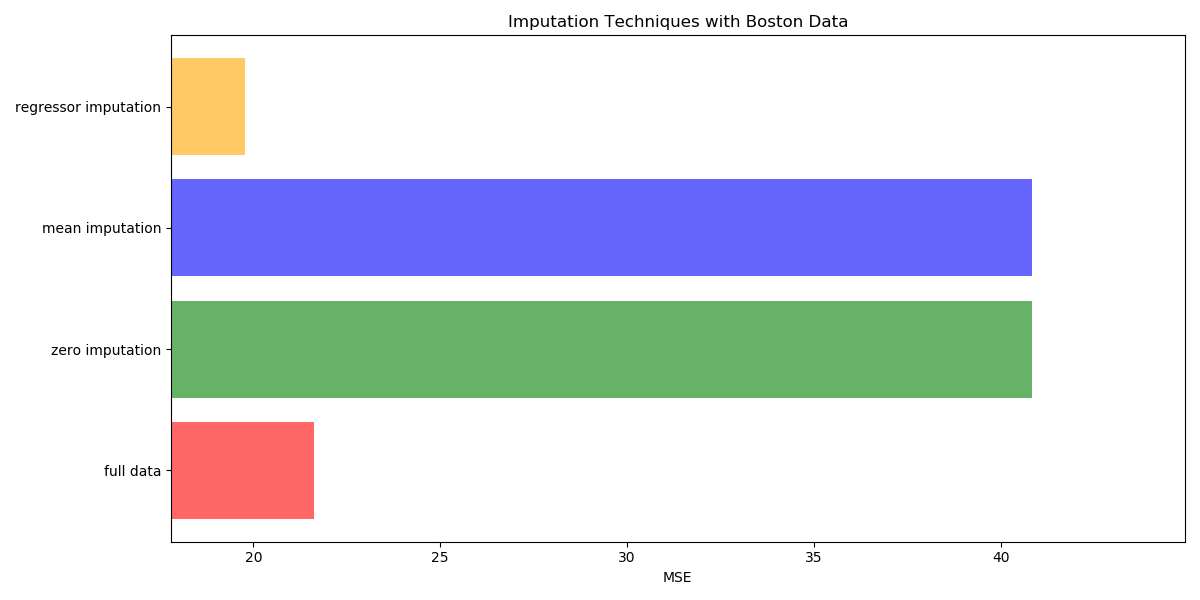# 五、机器学习中调参的基本思想

用来衡量模型在未知数据上的准确率的指标，叫做泛化误差（Genelization error）

当模型在未知数据（测试集或者袋外数据）上表现糟糕时，我们说模型的泛化程度不够，泛化误差大，模型的效果 不好。泛化误差受到模型的结构（复杂度）影响。看下面这张图，它准确地描绘了泛化误差与模型复杂度的关系， 当模型太复杂，模型就会过拟合，泛化能力就不够，所以泛化误差大。当模型太简单，模型就会欠拟合，拟合能力 就不够，所以误差也会大。只有当模型的复杂度刚刚好的才能够达到泛化误差最小的目标。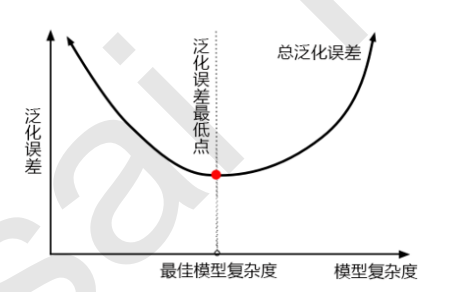1）模型太复杂或者太简单，都会让泛化误差高，我们追求的是位于中间的平衡点
2）模型太复杂就会过拟合，模型太简单就会欠拟合
3）对树模型和树的集成模型来说，树的深度越深，枝叶越多，模型越复杂
4）树模型和树的集成模型的目标，都是减少模型复杂度，把模型往图像的左边移动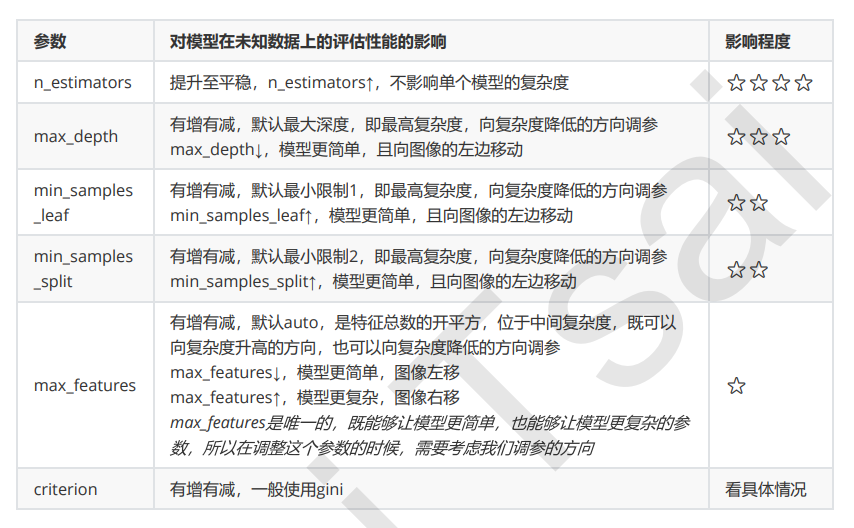# 六、 实例：随机森林在乳腺癌数据上的调参

from sklearn.datasets import load_breast_cancer
from sklearn.ensemble import RandomForestClassifier
from sklearn.model_selection import GridSearchCV
from sklearn.model_selection import cross_val_score
import pandas as pd
import numpy as np
from matplotlib import pyplot as plt

#print(x, y)
x = breast.data
y = breast.target

#单次实验结果
rfc = RandomForestClassifier()
score = cross_val_score(rfc, x, y, cv=10).mean()
print(score)  #0.9578320802005011

#画n_estimator学习曲线，选取最佳参数值
scores = []
for i in range(0, 200, 10):
rfc = RandomForestClassifier(n_estimators=i+1, n_jobs=-1, random_state=0)
#做迭代循环的时候，一定要记得加n_jobs=-1这个参数
score = cross_val_score(rfc, x, y, cv=10).mean()
scores.append(score)
#random_state=0时 0.9649122807017545 111
#random_state=90时 0.9631265664160402 71

print(max(scores), scores.index(max(scores)) * 10 + 1)
plt.figure(figsize=(16, 8))
plt.plot(range(1, 201, 10), scores)
plt.show()

#选定了random_state=0时 0.9649122807017545 111这个结果，我们可以将范围缩小到105到115之间，选定准确的数值
scores = []
for i in range(105, 116):
rfc = RandomForestClassifier(n_estimators=i, n_jobs=-1, random_state=0)
#做迭代循环的时候，一定要记得加n_jobs=-1这个参数
score = cross_val_score(rfc, x, y, cv=10).mean()
scores.append(score)
print(max(scores), [*range(105, 116)][scores.index(max(scores))])
#0.9666666666666666 110  #将n_estimators设置为110
plt.figure(figsize=(16, 8))
plt.plot(range(105, 116), scores)
plt.show()

#网格搜索，书写网格搜索的参数
#max_depth
param_grid = {'max_depth': np.arange(1, 20, 1)}
# 一般根据数据的大小来进行一个试探，乳腺癌数据很小，所以可以采用1~10，或者1~20这样的试探
# 但对于像digit recognition那样的大型数据来说，我们应该尝试30~50层深度（或许还不足够
#  更应该画出学习曲线，来观察深度对模型的影响
rfc = RandomForestClassifier(n_estimators=110, random_state=0)
GS = GridSearchCV(rfc,param_grid,cv=10)
GS.fit(x, y)
print(GS.best_params_)
print(GS.best_score_) #0.9666666666666666

#score没有变化，说明max_depth调参没有用诶，已经在最大值了

#min_samples_split
param_grid = {'min_samples_split': np.arange(2, 2+20, 1)}
rfc = RandomForestClassifier(n_estimators=110, random_state=0)
GS = GridSearchCV(rfc, param_grid, cv=10)
GS.fit(x, y)
print(GS.best_params_) #{'min_samples_split': 2}
print(GS.best_score_)  #0.9666666666666666
#说明调参没有用···

#min_samples_leaf
param_grid = {'min_samples_leaf': np.arange(1, 1+10, 1)}
rfc = RandomForestClassifier(n_estimators=110, random_state=0)
GS = GridSearchCV(rfc, param_grid, cv=10)
GS.fit(x, y)
print(GS.best_params_) #{'min_samples_leaf': 1}
print(GS.best_score_)  #0.9666666666666666 已经是最大深度了

#max_features
'''
param_grid = {'max_features': np.arange(5, 30, 1)}
rfc = RandomForestClassifier(n_estimators=110, random_state=0)
GS = GridSearchCV(rfc, param_grid, cv=10)
GS.fit(x, y)
print(GS.best_params_) #{'max_features': 7}
print(GS.best_score_)  #0.968421052631579
'''

#调整Criterion
param_grid = {'criterion': ['gini', 'entropy']}
rfc = RandomForestClassifier(n_estimators=110, random_state=0, max_features=7)
GS = GridSearchCV(rfc, param_grid, cv=10)
GS.fit(x, y)
print(GS.best_params_)  #{'criterion': 'entropy'}
print(GS.best_score_)  #0.9719298245614036

#最终参数为n_estimators=110, random_state=0, max_features=7, criterion=entropy
rfc = RandomForestClassifier(n_estimators=110, random_state=0, max_features=7, criterion='entropy')
score = cross_val_score(rfc, x, y, cv=10).mean()
print(score)
#0.9719298245614036

展开全文机器学习 sklearn python
• 随机森林标准手写数据分类 介绍 随机森林，指的是利用多棵树对样本进行训练并预测的一种分类器，将许多棵决策树整合成森林，并合起来用来预测最终结果，可以用来做分类、回归等问题。大多数情况下效果远要比SVM...

## 随机森林对标准手写数据集分类

### 介绍

随机森林，指的是利用多棵树对样本进行训练并预测的一种分类器，将许多棵决策树整合成森林，并合起来用来预测最终结果，可以用来做分类、回归等问题。大多数情况下效果远要比SVM，log回归，KNN等算法效果好。

### 随机森林的构建过程

1.从原始训练集中随机有放回采样选出m个样本，共进行N次采样，生成N个训练集
2.对于N个训练集，我们分别训练N个决策树模型
3.对于单个决策树模型，假设训练样本特征的个数为n，那么每次分裂时根据信息增益/信息增益比/基尼指数选择最好的特征进行分裂
4.每棵树都一直这样分裂下去，直到该节点的所有训练样例都属于同一类。在决策树的分裂过程中不需要剪枝
5.将生成的多棵决策树组成随机森林。对于分类问题，按多棵树分类器投票决定最终分类结果，对于回归问题，由多棵树预测值的均值决定最终预测结果

### 优点、缺点

具有极高的准确率
随机性的引入，使得随机森林不容易过拟合
随机性的引入，使得随机森林有很好的抗噪声能力
能处理很高维度的数据，并且不用做特征选择
既能处理离散型数据，也能处理连续型数据，数据集无需规范化
训练速度快，可以得到变量重要性排序
容易实现并行化
当随机森林中的决策树个数很多时，训练时需要的空间和时间会较大
随机森林模型还有许多不好解释的地方，有点算个黑盒模型

from sklearn.ensemble import RandomForestClassifier
data=[[0,0,0],[1,1,1],[2,2,2],[1,1,1],[2,2,2],[3,3,3],[1,1,1],[4,4,4]]
target=[0,1,2,1,2,3,1,4]
rf = RandomForestClassifier()
rf.fit(data,target)
RandomForestClassifier(bootstrap=True, class_weight=None, criterion='gini',
max_depth=None, max_features='auto', max_leaf_nodes=None,
min_samples_leaf=1, min_samples_split=2,
min_weight_fraction_leaf=0.0, n_estimators=10, n_jobs=1,
oob_score=False, random_state=None, verbose=0,
warm_start=False)
print(rf.predict_proba([[1,1,1]]))
#[[ 0.  1.  0.  0.  0.]]
#输出是5个数，因为target有5个值

import matplotlib.pyplot as plt
import matplotlib.pyplot
import seaborn as sns
from sklearn.metrics import confusion_matrix
from sklearn.model_selection import train_test_split
from sklearn.ensemble import RandomForestClassifier
from sklearn import metrics
fig = plt.figure(figsize=(6,6))
fig.subplots_adjust(left=0, right=1, bottom=0, top=1, hspace=0.05, wspace=0.05)
for i in range(64):
ax.imshow(digits.images[i], cmap=plt.cm.binary, interpolation='nearest')
ax.text(0,7,str(digits.target[i]))
x_train, x_test, y_train, y_test = train_test_split(digits.data, digits.target, random_state=0)
model = RandomForestClassifier(n_estimators=1000)
model.fit(x_train, y_train)
ypre = model.predict(x_test)
print(metrics.classification_report(ypre, y_test))
mat = confusion_matrix(y_test, ypre)
sns.heatmap(mat.T, square=True, annot=True, fmt='d', cbar=False)
plt.xlabel('true label')
plt.ylabel('predicted label')
matplotlib.pyplot.show()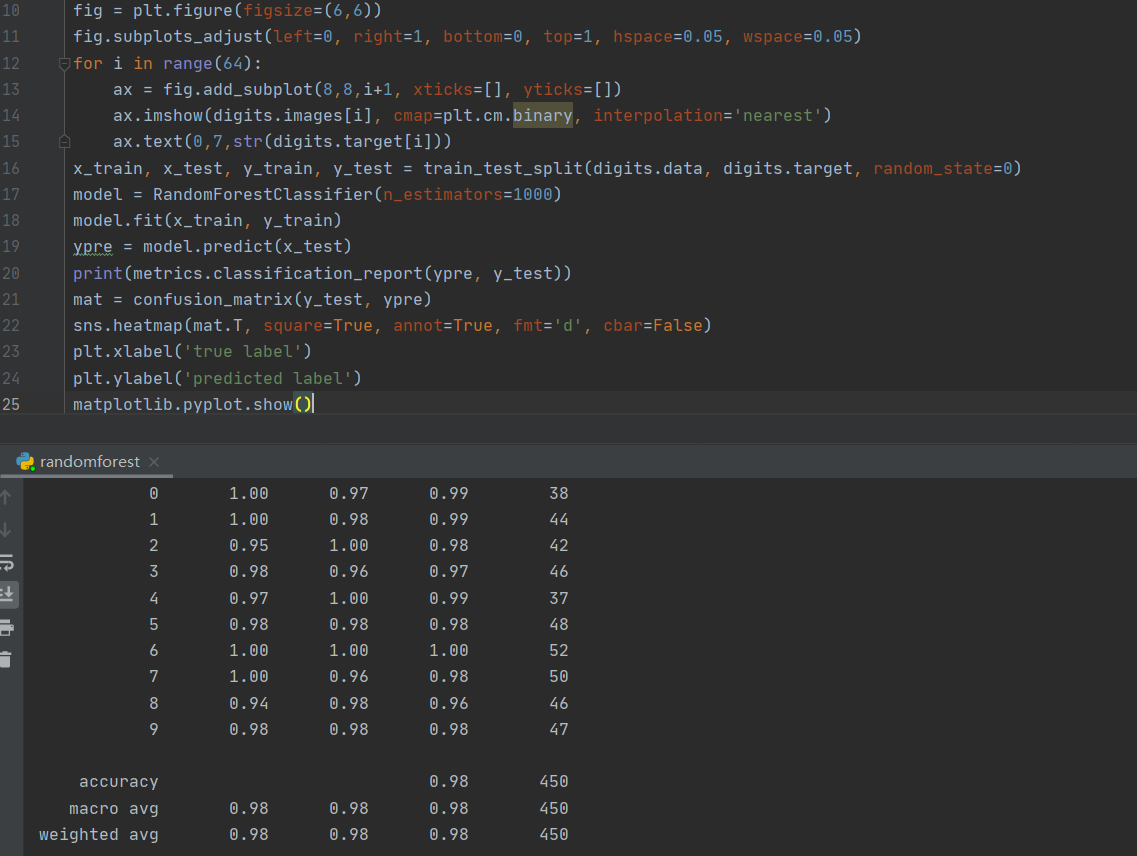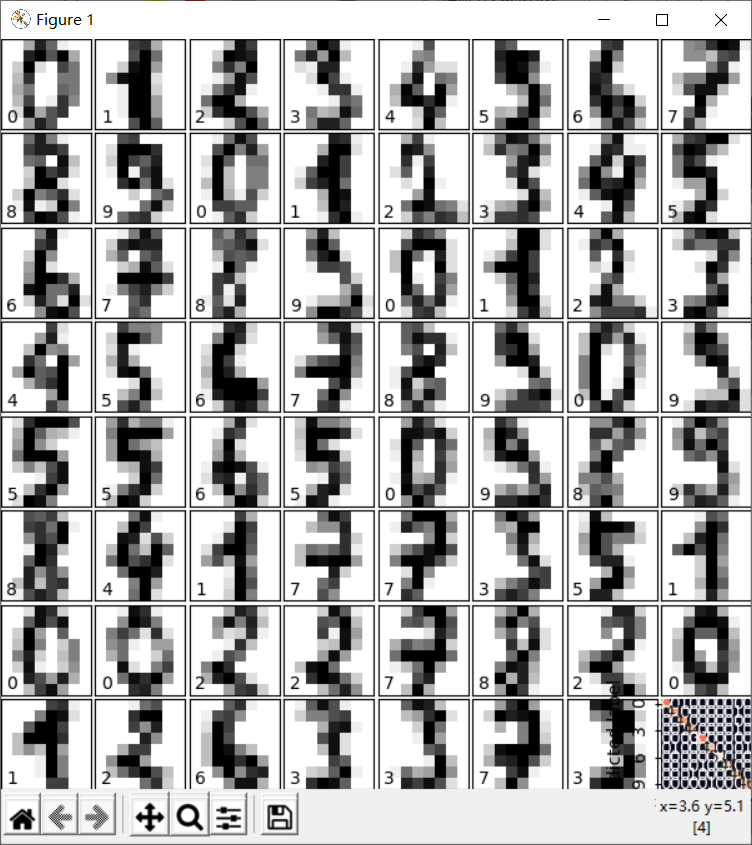展开全文机器学习
• 因此，为了统一比较的标准，保证结果的可靠性，我们在分析数据之前，需要对原始变量进行一定的处理，即本篇将向大家介绍的数据标准化处理，将原始数据转化为无量纲、无数量级差异的标准化数值，消除不同指标之间因...机器学习 中心化
• #实例一个随机森林分类器对象 指定包含5棵决策树，最大叶子节点数为16，用5个线程进行训练 rnd_clf = RandomForestClassifier(n_estimators=15, max_leaf_nodes=20, n_jobs=5) rnd_clf.fit(X_train, y_train) y_...机器学习 python 决策树
• 一、决策树(类型、节点特征选择的算法原理、优缺点、随机森林算法产生的背景)1、分类树和回归树由目标变量是离散的还是连续的来决定的；目标变量是离散的，选择分类树；反之(目标变量是连续的，但自变量可以是分类的...
• 说明：这是一个机器学习实战...某电器公司的燃气灶产品销售额一直在国内处于领先地位，把产品质量视为重中之重，每年都要对其产品质量数据进行分析研究，以期不断完善，精益求精。 2.获取数据 本次建模数据来源于...python 机器学习 项目实战
• 随机森林 随机森林的生成算法 随机森林的随机性 随机森林的优势 随机森林的参数详解 随机森林模型提高方法 最大特征数（max_features） 子树的数量（n_estimators）最重要的 最大深度（max_depth） 内部...机器学习 集成学习
• 阅读报告-A random forests...4.观察到虽然BRF显著改善了少数类的分类，而且优于普通的随机森林算法，在标准不平衡数据集上其性能也与RFQ算法大致相同。但在高复杂性，高不平衡性和高维度的不平衡数据集中处于劣势。分位数
• 公众号：尤而小屋作者：Peter编辑：Peter大家好，我是Peter~新年的第一个项目实践~给大家分享一个新的kaggle案例：基于随机森林模型（RandomForest）的心脏病人预测...python 机器学习 人工智能
• 随机森林图像matlab代码搜索九号星球 欢迎来到第九行星研究资料库！ 搜索使用来自的数据，并着眼于距地球约200AU的跨海马物体。 这是我在2017年夏季为该项目编写的一些代码。 专案 轨迹模拟 位置预测 候选人评估网站...
• 2019独角兽企业重金招聘Python工程师标准>>> ...
• 基于树的算法，如决策树（Decision Tree）、随机森林(Random Forest)和GBDT，xgboost等，对变量的大小不敏感。因此，在拟合这类模型之前，不需要进行标准化。 以决策树使用信息熵进行特征区间的划分为例，对于特征...人工智能 深度学习 机器学习 决策树
• 采用了随机森林和支持向量机的方法进行实验。对原始数据进行了异常值处理和属性数字的预处理操作，得到实验数据。实验1对数据进行十折交叉验证取十次结果平均值作为最终结果，度量方法为准确率，分别在两种模型上...支持向量机
• 文章目录前言一、使用决策树和随机森林数据分类二、代码拆分成训练数据和测试数据CART决策树分类检查准确率检查准确率三、总结 前言 在研究机器学习，发现了一些宝藏算法，写下来让自己记住，超有用！同时也想要...机器学习 python 深度学习 神经网络
• 使用随机森林算法完成基本建模任务∶包括数据预处理、特征展示、完成建模并进行可视展示分析。 分析数据样本量与特征个数对结果的影响∶在保证算法一致的前提下，增加数据样本个数，观察结果变算法 python 机器学习 人工智能
• 机器学习 随机森林分类Tree-based algorithms are popular machine learning methods used to solve supervised learning problems. These algorithms are flexible and can solve any kind of problem at hand ...算法 决策树 python 机器学习 人工智能
• 集成算法概述 集成学习（ensemble learning）是时下非常流行的机器学习...在现在的各种算法竞赛中，随机森林，梯度提升树（GBDT），Xgboost等集成 算法的身影也随处可见，可见其效果之好，应用之广。 集成算法的目标机器学习 sklearn
• 1、对数据进行处理并训练评估模型 from sklearn.model_selection import train_test_split, cross_val_score, KFold, GridSearchCV import pandas as pd import numpy as np from sklearn.ensemble import ...
•神经网络 机器学习 pythonpython
• 研究大纲 介绍数据集和研究的目标 探索数据集 可视 使用Chi-Square独立检验、Cramer's V检验和GoodmanKruskal tau值对数据进行探索 ... 预测模型，Logisitic... 随机森林模型 用RandomForest和Logisit..R语言 逻辑回归
• 1、背景介绍 单棵决策树的劣势 有时候单棵决策树可能难以实现较高的准确率，这主要是由以下几个方面决定的： 求解一棵最优（泛化误差最小）的决策树是一个NP难（无法穷极所有... 随机森林是一种以决策树为基分类...机器学习
• Kaggle案例：基于随机森林的心脏病人预测分类 大家好，我是Peter~ 今天给大家分享的一个kaggle案例：基于随机森林模型（RandomForest）的心脏病人预测分类。本文涉及到的知识点主要包含： 数据预处理和类型转化 ...机器学习
• ## Python 中的随机森林

千次阅读 2022-01-04 22:27:15
什么是随机森林 众所周知，树模型是高方差、低偏差的模型。因此，它们容易过度拟合训练数据。如果我们不修剪树模型或引入早期停止标准（例如每个叶节点的最小实例数），我们可以概括一下树模型的作用，这很吸引人...python 机器学习
• NSL-KDD 基于随机森林分类模型 数据集 NSL-KDD数据集是网络安全领域相对权威的入侵检测数据集，它对KDD 99的一些固有问题做了改进。 （1）NSL-KDD数据集的训练集和测试集中不包含冗余记录，使检测更加准确。 （2）...网络安全
• 该项目全流程通过Python实现，对泰坦尼克号幸存者数据进行了专业全面的数据挖掘工作，包括数据清洗、特征工程、降维可视、构建随机森林模型、调参可视、绘制学习曲线、绘制ROC曲线和PR曲线等..python sklearn 机器学习
• 随机森林 单颗决策树缺点：易过拟合的缺点 传统机器学习处理过拟合通常采用集成学习 (多颗树投票) 随机森林的生成方法【在bagging的基础上+CART树】： 1.从总数为N样本集中通过重采样的方式产生n个样本 ...决策树 机器学习...